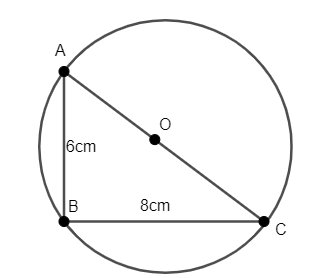QUESTION

# The radius of the circle, passing through the vertices of a right-angled triangle when the lengths of perpendicular sides are 6cm and 8cm is(a) 10cm(b) 14cm(c) 7cm(d) 5cm

Hint: Find the length of the hypotenuse of the right-angled triangle using Pythagorean Property. Use the fact that if the circle has to pass through the vertices of a triangle, the diameter of the circle must be equal to the length of the hypotenuse of the triangle. Divide the hypotenuse by 2 to calculate the radius of the circle.

Let’s assume that $\Delta ABC$ is the right-angled triangle, right-angled at B and the centre of the circle is O, as shown in the figure.So, we will now calculate the length of hypotenuse use Pythagorean Property. In a right-angled triangle $\Delta ABC$ right angled at B, we have ${{\left( AB \right)}^{2}}+{{\left( BC \right)}^{2}}={{\left( AC \right)}^{2}}$.
Substituting $AB=6cm,BC=8cm$ in the above equation, we have ${{6}^{2}}+{{8}^{2}}={{\left( AC \right)}^{2}}$.
Thus, we have ${{\left( AC \right)}^{2}}=36+64=100$. Taking square root on both sides, we have $AC=\sqrt{100}=10cm$. Thus, the diameter of the circle is 10cm.
Thus, the radius of the circle $=\dfrac{10}{2}=5cm$.
Note: We can use the fact that if the circle has to pass through the vertices of a right-angled triangle, then the diameter of the circle must be equal to the length of the hypotenuse of the triangle because $\angle ABC$ is an angle within a semicircle.